# Simulink and MATLAB objects

Recently, I spoke with a user who needed to interface a Simulink model with an external flight simulator implemented as java classes. He was able to exchange data with his simulator at the MATLAB prompt, but was not sure how to make that happen in Simulink.

Here is what I proposed.

The problem

Let's illustrate the problem with a very simple example using the Date class available in the java.util package. If you never worked with Java Libraries in MATLAB, you can get an overview in this section of the documentation

In MATLAB, I can create a java.util.Date object: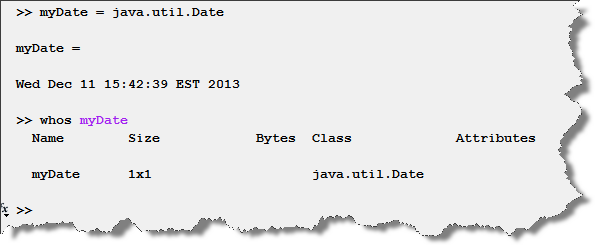Once the object is in the base workspace, I can call any of its method: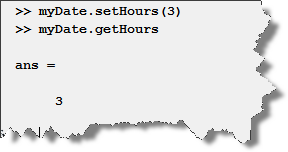This is simple... but in Simulink how to create the object once and access its methods every step?

The solution: A persistent object in the MATLAB Function Block

One easy way to implement something like that is using the MATLAB function block.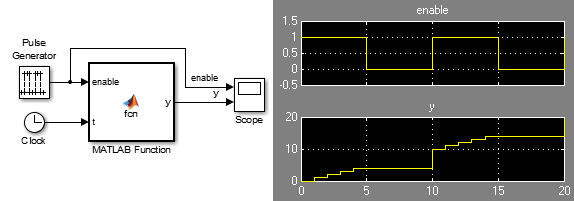The MATLAB Function block works by converting MATLAB code to C code and executing it during the simulation. The MATLAB function block can only generate code for a subset of the MATLAB language. However, it can call outside the generated C code, back to MATLAB, to run the expressions that do not support code generation. These functions are referred to as extrinsic functions, as they execute outside the generated C code. This is useful if your block will only be used for simulation.

When I use the MATLAB Function block for simulation only applications like this one (I do not need to generate code for an embedded system), I like to make all my code extrinsic. That way I do not need to worry about MATLAB Code Design Considerations for Code Generation.

To do this, I create a separate MATLAB function file and make it extrinsic. The code in the MATLAB Function block looks like: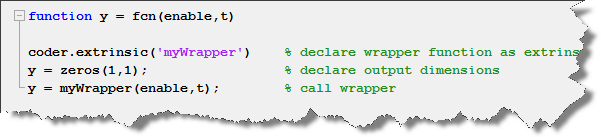In the extrinsic function myWrapper.m, I manage the object and implement my algorithm. To keep the object properties from step to step, I make it persistent, and I initialize it only once using the isempty function: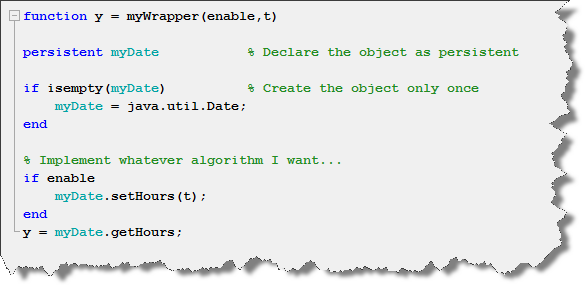and that's it... I can now access all the methods of this object and its properties remain persistent from step to step.

Now it's your turn

Note that this is not the only way to implement such functionality. A MATLAB Level 2 S-Function would also give similar results.

Give that a try and let us know what you think by leaving a comment here.

|

### Comments

To leave a comment, please click here to sign in to your MathWorks Account or create a new one.